## Off Topic: A geometry problemStarted by 6 years ago30 replieslatest reply 6 years ago212 views
Hi Troops.  Forgive me for posting a non-DSP topic here, but I simply had to show the following to someone. I was looking at my grandson' high school "Common Core" geometry book. In that book I found the following geometry "Example Problem." Does that problem's solution bother you folks as much as it bothers me?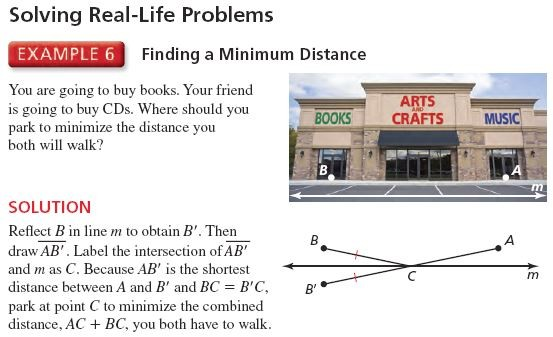Actually, the given method for solving this problem does more than just bother me, it scares me.

 "Geometry", written by Ron Larson and Laurie Boswell, published by Big Ideas Learning LLC. See page 189 of PDF file at: http://www.bigideaslearning.com/for-reviewers/texas/geo_pe/hstx_geometry_pe_04.pdf

[ - ]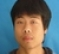I remember I came across this problem at Grade 7. That is exactly how we taught to solve the problem. Find the solution very intuitive. :)

[ - ]Given the unrestricted definition of this problem I would like to park in the arts and crafts store giving both AB

[ - ]KISS = Keep it Simple Silly.

This problem solving reflects somebody has lost their natural way of thinking and relies heavily on copycat pseudo-scientific approach.

[ - ]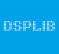We can just write AC+BC as function of C point.

AC =sqrt(h^2+ (1-C)^2)

BC = sqrt(h^2 + C^2)

Here h is distance between line m and points A and B, C is C point coordinate from 0 to 1.

Minimum will be if Derivative AC+BC corresponds to C will be 0.

From direvative equation we will have that 1-C =C.So C =0.5

[ - ]Hi dsplib.  I agree with you.  If you're interested in my solution, just scroll down in this thread.

[ - ]Hmmmmmm.  I don't think it is as bad as you say.  Had they shown an additional different position for 'C', perhaps several of them, why this suffices as a "proof" would be clearer.

On the other hand, getting a bit persnickety, depending on who is driving, whether you pull in forward or back in would have a larger impact on the total walking distance than the choice of parking spaces between the two stores.

For extra credit, assume your friend walks slower and there are many more parking spaces between the stores, find the space that minimizes the combined walking time.

Ced
[ - ]Hi.  Are you saying there is more than one correct answer to this minimization problem?

[ - ]Hi Rick,

No.

The first paragraph is the same sentiment as SteveSmith's comment below.  Showing alternatives to the final solution would better show why the final solution is optimal.

The second paragraph is a somewhat obtuse commentary that the mathematical model isn't a very good fit of the physical situation.  Not to mention the difference between the discrete range of real world answers (individual parking spaces) versus the continuous nature of the mathematical model.  The discrete vs continuous case is very on topic for DSP discussions.

The third paragraph is an allusion to the derivation of Snell's Law.

As a side note, the solution reminds me of the "mirror trick" you can use when aiming a bank shot in pool.

I was hoping you could clarify why you say the book's solution is wrong.

Ced
[ - ]Hi.  If you're interested in my solution, just scroll down in this thread.

[ - ]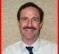If you are driving the car and your friend is the passenger then this is the correct solution.  However, what if your friend was driving?  And shouldn't the book-buyer be closer because he or she will have heavier things to carry back to the car?  And did you ever wonder about those people who jockey to park closer to the entrance at the health club?

Seriously, I don't see anything wrong with this as a purely geometric solution.  It's not the best solution to this problem but they aren't teaching trigonometry in this chapter and it illustrates the concepts of mirroring and straight lines.

[ - ]Hi Greg.  In my opinion, the only thing wrong with the geometry book's given solution is that it is incorrect.  If you're interested in my solution, just scroll down in this thread.

[ - ]Rick,

Geometry was never my strong suit, but the solution seems correct to me.  They do make one error in wording by saying that they "minimize the combined distance, AC + BC, you both have to walk."  I interpreted it as "each person walking the same distance."  If that's right I suppose it should have been worded to minimize the distance that each of you have to walk.

Greg

[ - ]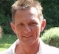If a compass and straight-edge solution is required, derivations are certainly not allowed, although I would tend to that approach too, since it's quite straight-forward.
The above solution doesn't come to my mind. Not even after quite a while trying to force it - though it's very smart.

My approach would have been this:
1. The solution must be independent of which is A or B, hence symmetric.
2. 1) means that there are only two possible solutions:
parking in the middle (in point C ) =2a)
or parking closest to B (in point Bm) =2b)
Draw both solutions 2a) and 2b).
3. with compasses draw a circle from B round Bm, thus giving the sum of both paths as straight line through A.
4. with compasses draw a circle from B round C, thus giving the sum of both paths as straight line through A.
5. circle round A through end of the now longer lines will show which is longer.
The other is the required solution.

Maybe too many circles and lines, but I would have found it in time :)

[ - ]The best solution is agreement of the two people and availability of parking spaces. Is this Forum alright? We might need to see Forum psychiatrist.

[ - ]Hi Kaz. The problem's question is clearly stated.  Unless I'm missing something here, there is one, and only one, correct answer for the location of point C. If you're interested in my solution, just scroll down in this thread.

[ - ]My idea to consider the right or left rim was overshoot:
Only thinking about a parking position far left from B or right from A makes clear that ways are always increasing. So minimum can only be in the middle.
But maybe that's not really geometry but ... what's it? Number theory?

[ - ]Hi bholzmayer.  If you're interested in my solution, just scroll down in this thread.

[ - ]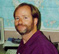Hey Rick!

Yes, it bothers me too, but it took me awhile to realize why... probably like everyone else.  That in itself says it's not a good problem for a basic geometry class.

What bothers me is that they answer the question in a different way than they ask it.

"Where should you park" implies that you consider all the points along m, evaluating how far each person walks from that point.  That is, for some point on m, which we can call x, the total distance walked is (B to x) + (x to A).  Then they should construct B', and show that (B' to x) is the same distance as (B to x).  Then you can jump to the conclusion that the shortest distance is a straight line between B' and A, resulting in the final answer that x = C.

But they don't do that... they immediately draw a straight line between B' and A, define the point C from that construction, and argue that C is the final answer.  But that procedure doesn't even bring in x, the other points that you need to consider as alternatives.

So, I think it is a correct solution, but a very poor learning example.

Thanks for giving us a distraction from the day!

[ - ]Hi Steve.  I was happy to read that Example 6 also bothered you.  If you're interested in my solution, just scroll down in this thread.

[ - ]Hi. I believe (1)this problem is a minimization problem, and (2)there is only one correct answer for the location of point C.

The book's solution to finding point C is not correct because the book's nonsensical method to arrive at a solution depends on where you draw line 'm'. If you lower the position of line 'm', from that given in the book's solution, you'll end up with a different location for point C. The book's method of solving this problem can produce multiple locations for point C, and that should immediately make you suspicious of the book's solution method.

Here's my solution: Draw points B and A (both lying on a horizontal line). Next, consider every point on the 2-dimensional plane and determine which points are equidistant from points B and A. Doing that, we pretty quickly realize that all the points equidistant from points B and A are on a line that I call "Line X" below: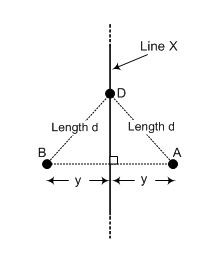For example, arbitrary point D on Line X is equidistant from points B and A.

Now, ...all we have to do is select the point C on Line X that minimizes the distance between point B and point C and minimizes the distance between point A and point C. I propose that the desired, and correct, location of point C is shown below: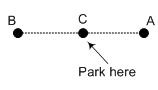[ - ]Hi Rick,

I think you have made a small mistake in your analysis of the book's solution.  If you move the line "m", you also move the point "B'" as it is supposed to be the reflection of "B" from line "m".  Thus the solution stays in the same place.  The book words it poorly, and IMO in the wrong sequence.

1) Place B'

2) Place C anywhere on the line

3) Recognize by symmetry (the lesson being taught) that CB' = CB.

4) Minimizing CB + CA is therefore the same as minimizing CB' + CA

5) CB' + CA is minimized when C lies on AB'

Your solution assumes that CA = CB, and you figure from there.  As the problem appears, that is true.  But suppose one of the stores is set back from the sidewalk further than the other.  In this case, the book solution will be correct and yours will not.

Ced

P.S. Kudos to you for being interested in your grandson's homework.
[ - ]Hi.  If we move line m, the book's solution will produce a new location for point C.  (In the book's solution, point C must always lie on line m.)

[ - ]Assuming the line is moved in a parallel manner, e.g. the sidewalk is wider or narrower.

Yes, it will move vertically on the diagram, but not horizontally.  It will still be halfway between the projection of A and B onto the line.

I'm not sure if you meant this or that it would move horizontally as well.

Ced
[ - ]Hi Ced.   On a 2-dimensional plane, you seem to be saying that the locations of the following two points, C and C', are equal to each other.  I think the locations of those two points are not equal to each other.[ - ]It would have been clearer had I originally said "Thus the solution stays in the same place horizontally."  or "Thus the solution stays in the same place on the line."  I tried to clarify that in my last response.  Your C to C' shifts vertically, but not horizontally (in terms of the diagram, not the 3D space of the problem).

Ced

[ - ]Hi Ced.  I'm sure you and I both understand this geometry problem and that we're actually in "agreement".  Did you see my most recent post down at the bottom of this thread?

[ - ]Rick, yes and yes.

If you look carefully at the photo, the line m is actually superimposed.  Poor color choice.

The problem is also poorly worded.  Taken literally, along the lines of ombz's answer, the car should be parked rammed into the Arts and Crafts store so the doors are flush with the storefront.  Then any placement right or left (between A and B) from wouldn't change the distance.

Ced

[ - ]Hi Rick.

So are you saying that the correct solution is to park the car in the middle of the arts and crafts store? :p

Guess the original question is simply missing to give a precise constraint on y. Hence the task was basically to "reason" in 2D but to give a 1D solution. Or in other words: only x can be determined exactly while for y we miss a 2nd constraint and the true solution space is not a point but a line. And it's the line perpendicular to AB such that the intersection with AB has equal distance to both A and B: the line that you draw above. On the other hand, the originally proposed solution is one way to get one possible solution within the solution space. So I don't see anything too wrong for that grade level except that it is not a well formulated question and an overcomplicated solution. I'd say teach these kids some common sense and let them play instead! :)

-Andy

[ - ]